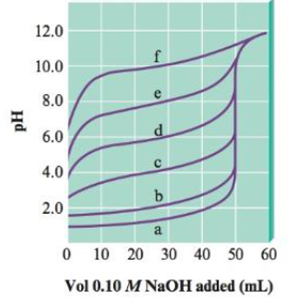# The following plot shows the pH curves for the titrations of various acids by 0.10 M NaOH (all of the acids were 50.0-mL samples of 0.10 M concentration). a. Which pH curve corresponds to the weakest acid? b. Which pH curve corresponds to the strongest acid? Which point on the pH curve would you examine to see if this acid is a strong acid or a weak acid (assuming you did not know the initial concentration of the acid)? c. Which pH curve corresponds to an acid with K a ≈ 1 × 10 −6 ?### Chemistry: An Atoms First Approach

2nd Edition
Steven S. Zumdahl + 1 other
Publisher: Cengage Learning
ISBN: 9781305079243

#### Solutions

Chapter
Section### Chemistry: An Atoms First Approach

2nd Edition
Steven S. Zumdahl + 1 other
Publisher: Cengage Learning
ISBN: 9781305079243
Chapter 14, Problem 90AE
Textbook Problem
7 views

## The following plot shows the pH curves for the titrations of various acids by 0.10 M NaOH (all of the acids were 50.0-mL samples of 0.10 M concentration).a. Which pH curve corresponds to the weakest acid?b. Which pH curve corresponds to the strongest acid? Which point on the pH curve would you examine to see if this acid is a strong acid or a weak acid (assuming you did not know the initial concentration of the acid)?c. Which pH curve corresponds to an acid with Ka ≈ 1 × 10−6?

(a)

Interpretation Introduction

Interpretation: The titration curve obtained after the reaction of various acids with NaOH is given. Various questions based on the given curve are to be answered.

Concept introduction: Titration is a quantitative chemical analysis method that is used for the determination of concentration of an unknown solution. In acid base titration, the neutralization of either acid or base is done with a base or acid respectively of known concentration. This helps to determine the unknown concentration of acid or base.

### Explanation of Solution

Explanation

The curve f has the highest pH and therefore, it belongs to weak acid.

The strength of an acid is determined by the value of pH . When the value of pH lies in between 07 , the given solution is acidic in nature. But when the value of pH lies in between 714 , the given solution is basic in nature

(b)

Interpretation Introduction

Interpretation: The titration curve obtained after the reaction of various acids with NaOH is given. Various questions based on the given curve are to be answered.

Concept introduction: Titration is a quantitative chemical analysis method that is used for the determination of concentration of an unknown solution. In acid base titration, the neutralization of either acid or base is done with a base or acid respectively of known concentration. This helps to determine the unknown concentration of acid or base.

(c)

Interpretation Introduction

Interpretation: The titration curve obtained after the reaction of various acids with NaOH is given. Various questions based on the given curve are to be answered.

Concept introduction: Titration is a quantitative chemical analysis method that is used for the determination of concentration of an unknown solution. In acid base titration, the neutralization of either acid or base is done with a base or acid respectively of known concentration. This helps to determine the unknown concentration of acid or base.

### Still sussing out bartleby?

Check out a sample textbook solution.

See a sample solution

#### The Solution to Your Study Problems

Bartleby provides explanations to thousands of textbook problems written by our experts, many with advanced degrees!

Get Started

Find more solutions based on key concepts
Both heart disease and cancer are due to genetic causes, and diet cannot influence whether they occur. T F

Nutrition: Concepts and Controversies - Standalone book (MindTap Course List)

Define the following terms: a. chromosome b. chromatin

Human Heredity: Principles and Issues (MindTap Course List)

Why does the expansion of a stars envelope make it cooler and more luminous?

Horizons: Exploring the Universe (MindTap Course List)

The gravitational force exerted on a solid object is 5.00 N. When the object is suspended from a spring scale a...

Physics for Scientists and Engineers, Technology Update (No access codes included)